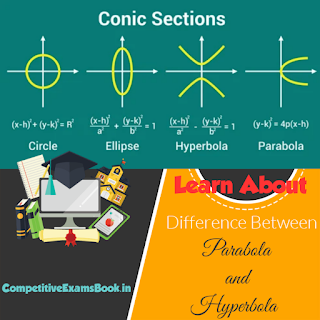News Breaking
Live
wb_sunny

## Breaking News

Learn About the Difference Between Parabola and Hyperbola

# Learn About the Difference Between Parabola and Hyperbola

Learn About the Difference Between Parabola and Hyperbola

The main distinction between a parabola and a hyperbola is their eccentricity. Eccentricity is equal to one for a parabola and greater than one for a hyperbola. Although they are both conic sections, there are several other characteristics that distinguish parabola and hyperbola.Learn About the Difference Between Parabola and Hyperbola
Let’s learn about the parabola and hyperbola in detail individually as well as understand the difference between the two curves.

What is Parabola?

A parabola is the section of a right circular cone by a plane parallel to the cone's generator. It is a point locus that moves so that the distance from a fixed point (focus) equals the distance from a fixed line (directrix).

Standard Equation of Parabola

In general, the standard Equation of Parabola is presented as

y² = 4ax,  when the directrix is parallel to the y-axis.

The standard parabola equation is, x² = 4ay if the parabola is sideways, i.e., the directrix is parallel to the x-axis.

In addition to these two, if the parabola is in the negative quadrants, the equations can be y² = -4ax and x² = -4ay.

Properties of Parabola

A parabola's important properties include the following:

• Eccentricity is a conic section factor that shows the roundness of the conic section. More eccentricity implies less spherical behaviour, whereas less eccentricity implies more spherical behaviour. It is represented by the letter e. The eccentricity of a parabola is the ratio of the distance between the focus and a point on the plane to the vertex and that point only. As a result, any parabola has an eccentricity of 1.
• The parabola is symmetric about its axis.
• The axis is perpendicular to the directrix.
• The axis connects the focus with the vertex.
• At the vertices, the tangent and directrix are parallel.
• The vertex is the focus's midpoint, where the directrix and axis intersect.
• If an is the distance between the focus and the vertex, the distance between the focus and the plane point is 2a, which is the same as the distance between the focus and the directrix. The axis of symmetry lies halfway along the latus rectum, indicating that the length of the latus rectum is 4a and the half is 2a. thanks

What is Hyperbola?

A hyperbola is a locus of points arranged in such a way that the distance between each focus is larger than one. In other words, the locus of a point travelling in a plane such that the ratio of its distance from a fixed point (focus) to a fixed line (directrix) is larger than one.

Standard Equation of Hyperbolae

When the centre of the hyperbola is at the origin and the foci are on the x- or y-axis, the equation of hyperbola is the simplest. A hyperbola's standard equation is as follows:

[(x² / a²) – (y² / b²)] = 1

where , b² = a² (e²  – 1)

Properties of a Hyperbola

Following are some of a hyperbola's key properties:

• Every hyperbola has two focal points, or foci. At each point on the hyperbola, the difference in the distances between the two foci is a constant.
• The hyperbola's two foci are connected by a straight line called the directrix, which is perpendicular to the conjugate axis of the hyperbola.
• The directrix and the transverse axis are always perpendicular.
• The transverse axis is where the foci and vertices are located.
• The tangent line to a hyperbola's directrix is always parallel to the hyperbola's vertices.
• The latus rectum measures 2b²/a in length for the hyperbola x²/a²-y²/b²=1.
• A hyperbola is considered to as rectangular or equilateral if the lengths of the transverse and conjugate axes are equal.

 What is the difference between Parabola and Hyperbola? Parabola Hyperbola A locus of points in a plane that are equally spaced out from a directrix or focus is known as a parabola. The difference of distances between a locus of points that are present in a plane to two fixed points and is a positive constant is how the hyperbola is defined. A parabola has a single point of focus and a directrix. A hyperbola has two foci and two directrices. Eccentricity, e = 1............................There are no asymptotes. Eccentricity, e>1..........................There are two asymptotes

Tags